# 978-0134292380 Chapter 4 Part 2

Document Type
Test Prep
Book Title
Fundamentals of Hydraulic Engineering Systems 5th Edition
Authors
A. Osman H. Akan, Ned H. C. Hwang, Robert J. Houghtalen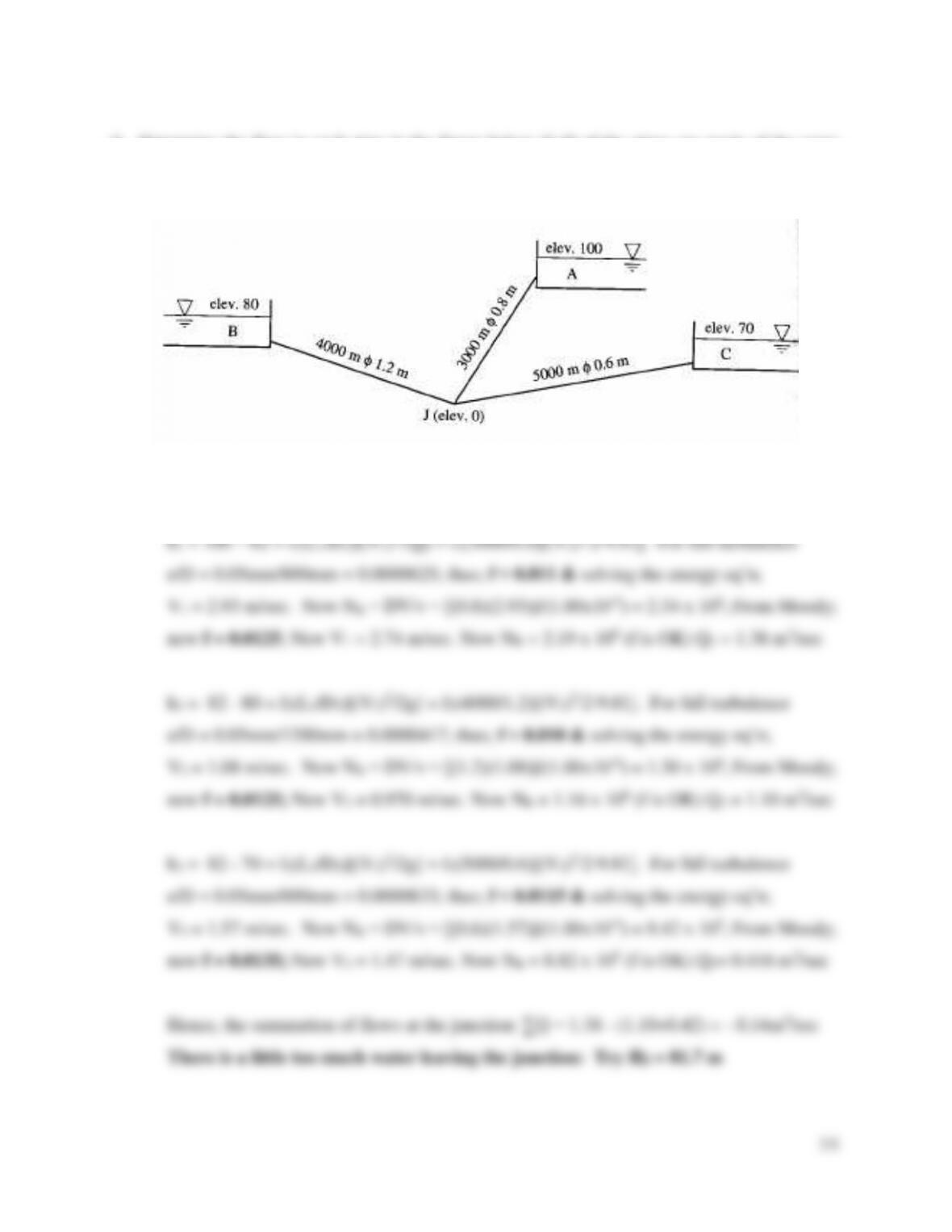36
9. Determine the flow in each pipe in the figure below if all of the pipes are made of the same
material with e = 0.05 mm and water temperature at 20°C = 1.00 x 10-6). Try an initial
energy level at the junction of 82 m, and go through one complete iteration of the solution
including a comment on your second trial energy level at the junction.
Ans. Assume a total energy elevation at the junction of HJ = 82 m. Then balance energy
between all reservoirs and the junction. (Use Darcy-Weisbach eq’n for friction loss.)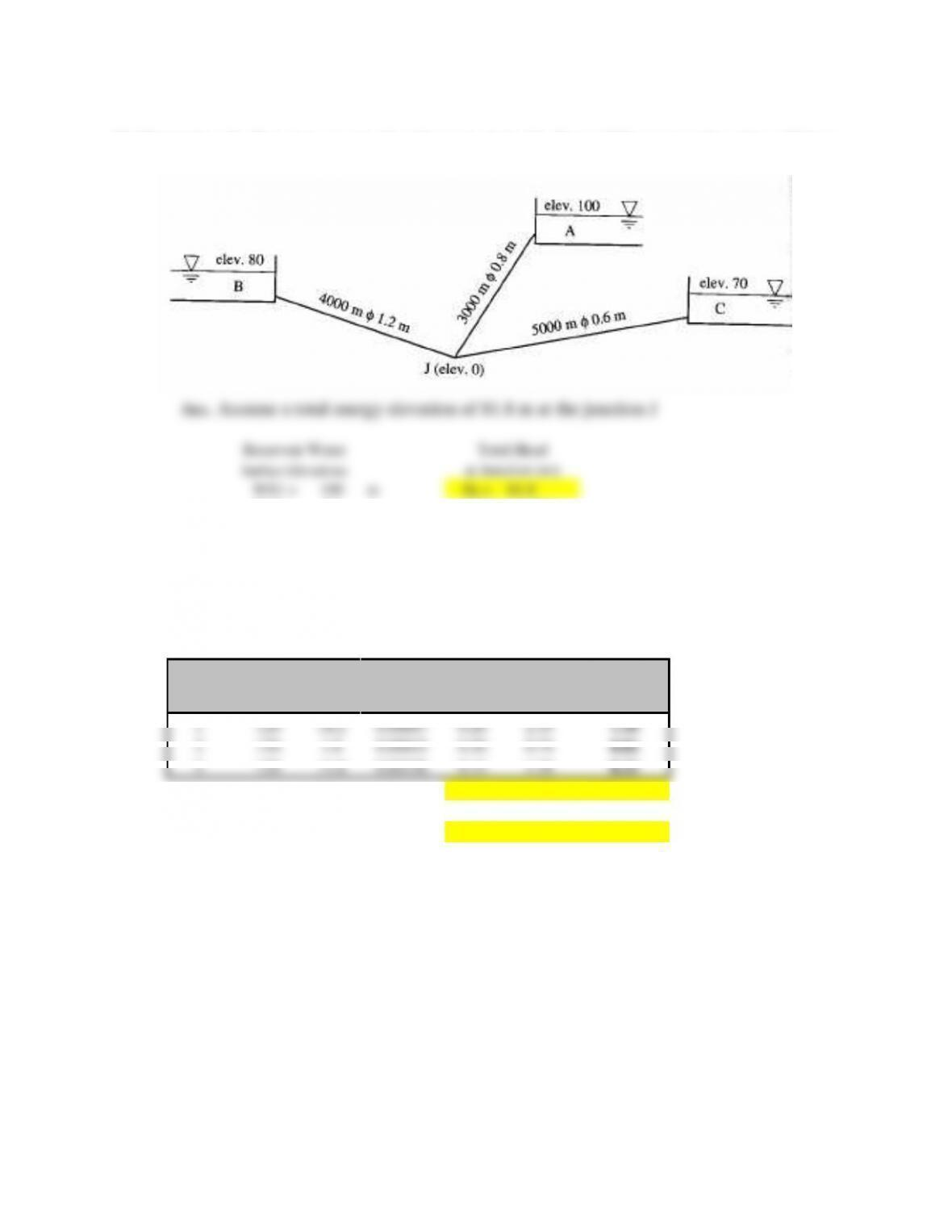10. Determine the flow into or out of each reservoir in the figure if the connecting pipes all have
a Hazen-Williams coefficient of 120. (Hint: Try a junction energy elevation of 81.8 m.)
81.8
WS2 =
80
m
Trial until ∑Qs
WS3 =
70
m
Balance
Pipe Lengths
Pipe Diameters
L1 =
3000
m
D1 =
0.80
L2 =
4000
m
D2 =
1.20
L3 =
5000
m
D3 =
0.60
Pipe#
CHW
hf
S*
Rh**
V***
Q
(m)
(m)
(m/sec)
(m3/sec)
1
18.2
0.00607
0.20
1.18
2
1.8
0.00045
0.30
0.74
0.84
3
11.8
0.00236
0.15
1.18
0.33
* S = hf/L (friction slope or EGL slope in this case)
If HJ < WSβ; ∑Q =
** Rh = D/4 (Equation 3.26)
*** V = 0.849CRh0.63S0.54 (Equation 3.27)
If HJ > WSβ; ∑Q =
11. A small branching pipe system distributes water to three holding tanks. Pipe 1 contains a
valve that is partly closed that limits the flow rate to 1.0 cfs with a valve head loss of 3.60
feet. Estimate the length of Pipe 2 given the following water surface (WS) elevation and pipe
data (lengths, diameters, and Hazen-Williams coefficients):
WS1 = 4020 ft L1 = 1000 ft D1 = 1.0 ft C1 = 80
WS2 = 4018 ft L2 = ? D2 = 10 in. C2 = 100
WS3 = 4000 ft L3 = 5000 ft D3 = 1.0 ft C3 = 120
The total energy elevation at the junction (HJ) is 4015 ft.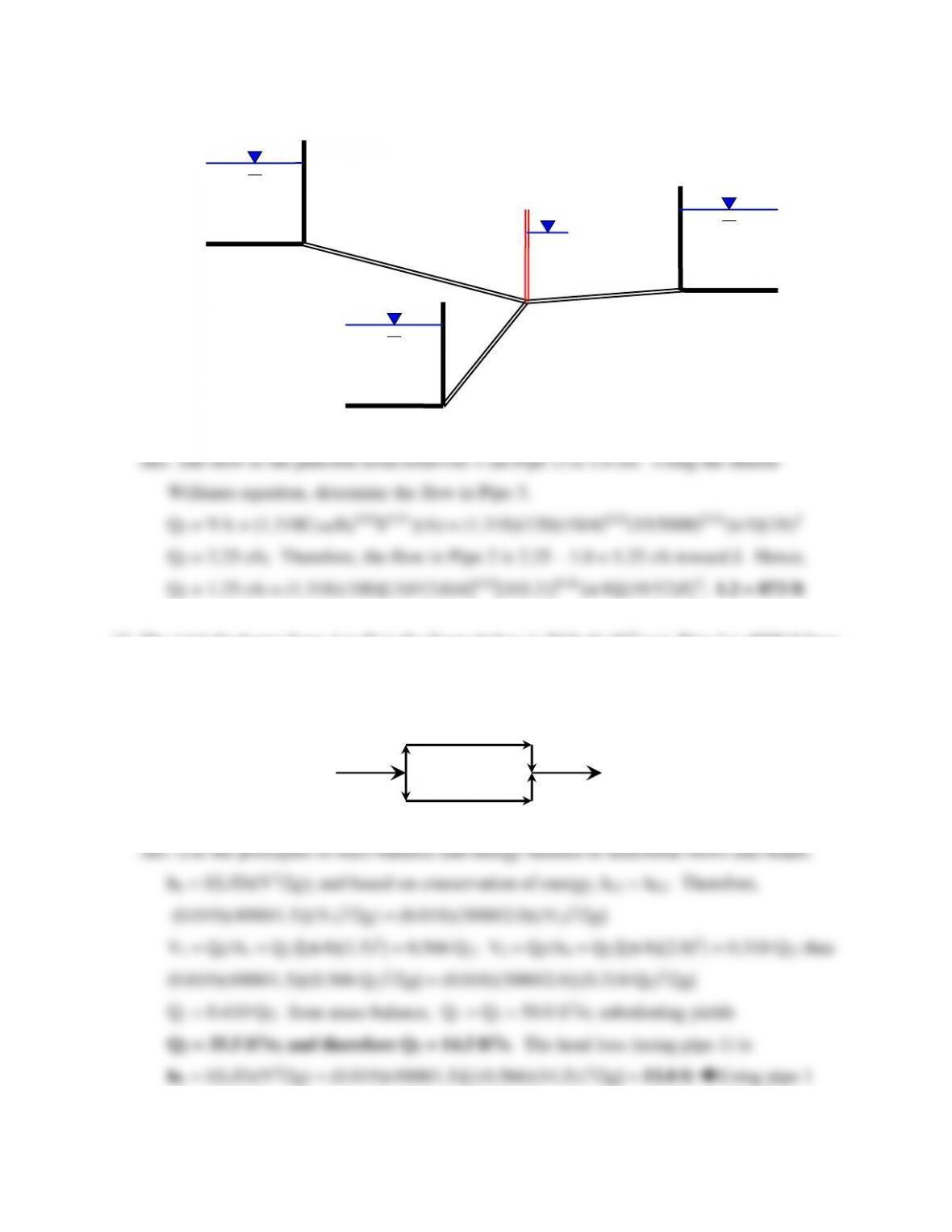38
12. The total discharge from A to B in the figure below is 50.0 cfs (ft3/sec). Pipe 1 is 4000 ft long
with a diameter of 1.5 ft, and pipe 2 is 3000 ft long with a diameter of 2 ft. Using Hardy-
Cross principles, determine the head loss between A and B and the flow rate in each pipe.
Assume f1 = 0.019, f2 = 0.018, ν = 1.08 x 10-5 ft2/sec, and ignore minor losses.
J
Pipe 2
Pipe 1
WS3
WS2
WS1
Reservoir
Reservoir 2
Reservoir 3
HJ
Pipe 3
1
2
B
A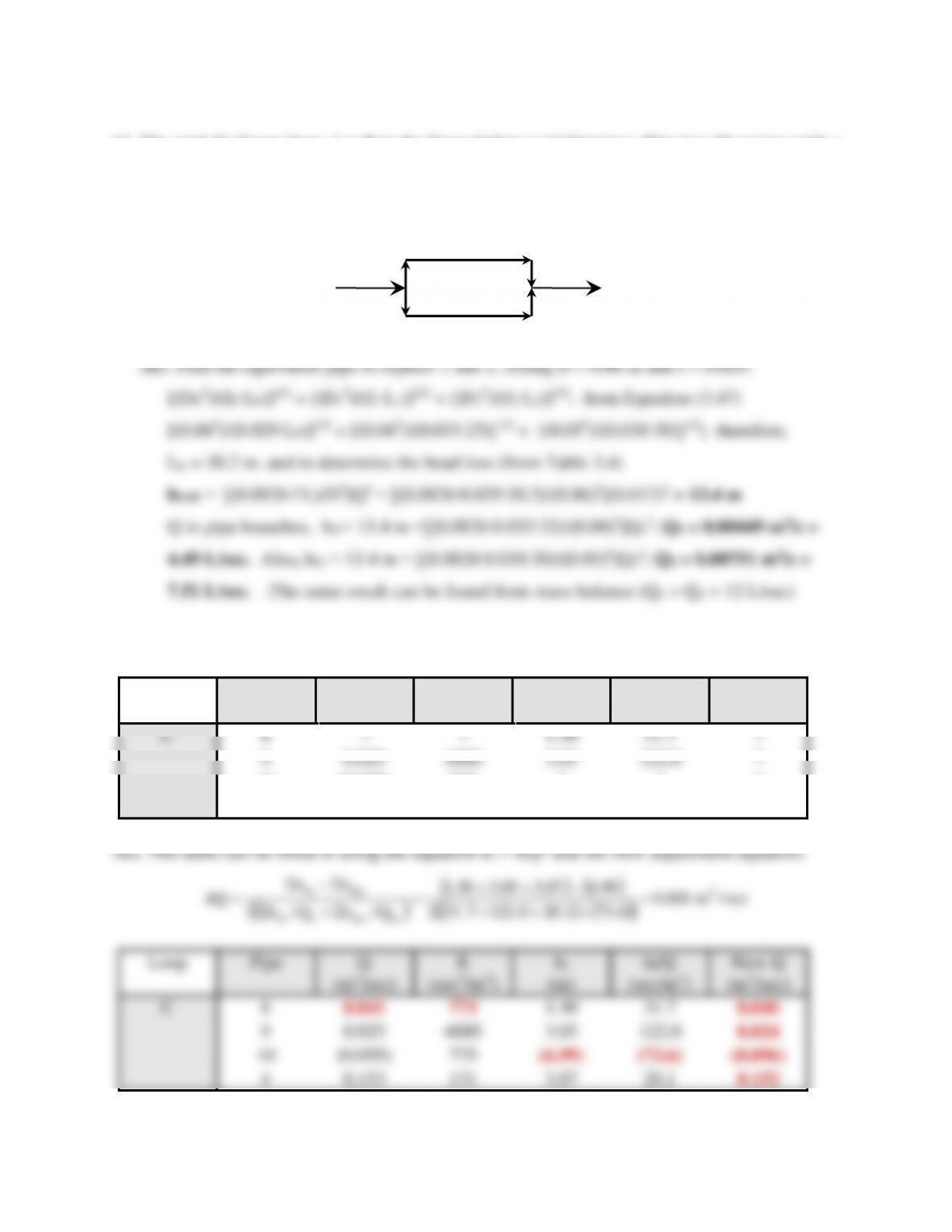39
13. The total discharge from A to B in the figure below is 12 liters/sec. Pipe 1 is 25 m long with a
diameter of 4 cm, and pipe 2 is 30 m long with a diameter of 5 cm. Using the method of
equivalent pipes, determine the head loss between A and B and the flow rate in each pipe.
Assume f1 = 0.033, f2 = 0.0γ0, ν = 1.00 x 10-6 m2/sec, ignore bend losses, and for the
equivalent pipe, let DE = 0.06 m and fE = 0.029.
14. Fill in the blanks for the partially completed pipe network solution table given below.
Loop
Pipe
Q
(m3/sec)
K
(sec2/m5)
hf
(m)
hf/Q
(sec/m2)
New Q
(m3/sec)
C
8
?
?
31.7
?
9
0.025
4880
122.0
?
10
(0.095)
775
?
?
?
4
0.153
131
3.07
20.1
?
1
2
B
A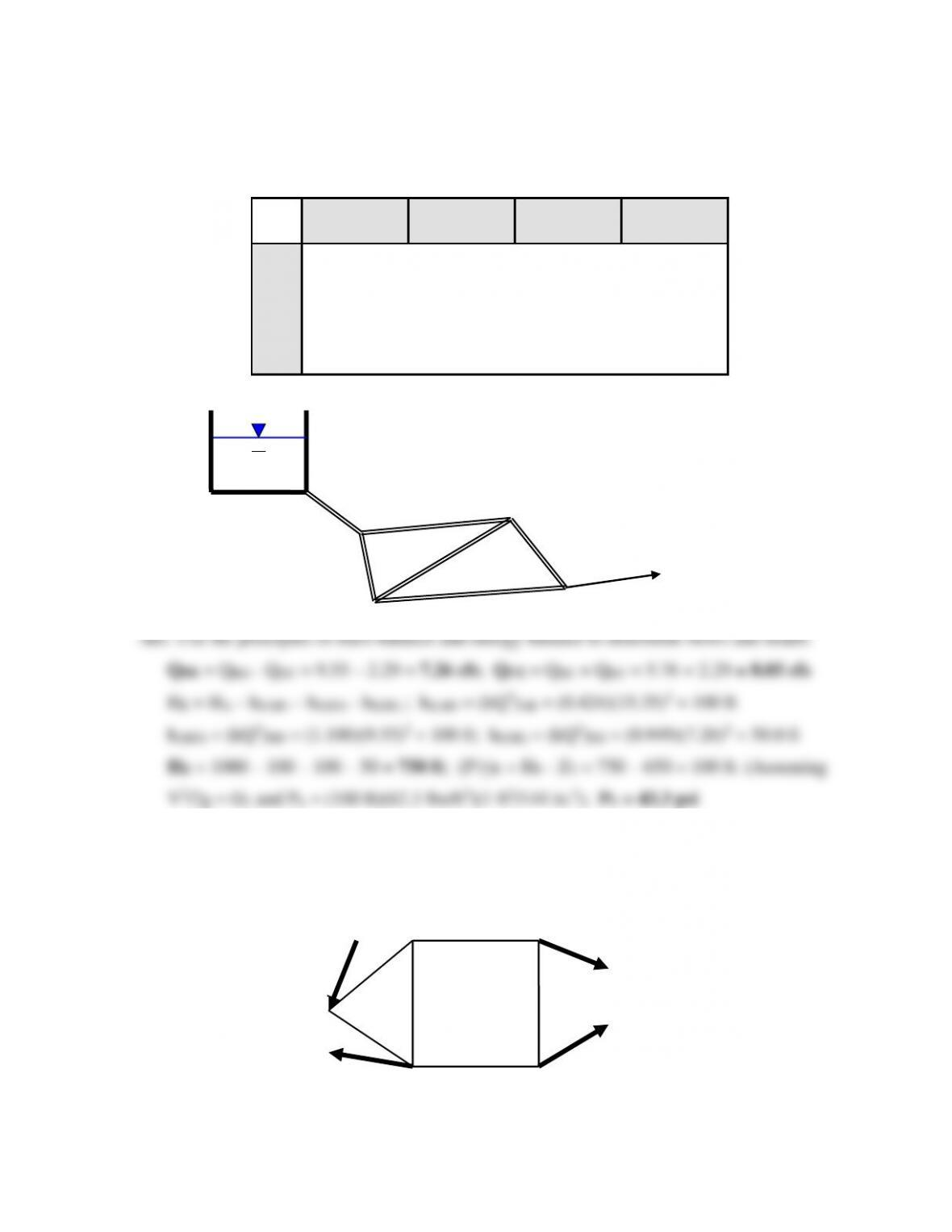40
15. From the pipe network data below, determine the flow in pipes CE and DE, and the pressure
head HE. Also determine the pressure (in psi) at E if the ground elevation is 650 ft.
Pipe
Flow
(ft3/sec)
Length
(ft)
Diameter
(in.)
K
(sec2/ft5)
AB
15.35
1000
12
0.424
BC
5.76
1500
8
1.510
BD
9.55
1200
12
1.100
DC
2.29
1500
6
9.530
CE
?
2000
12
1.540
DE
?
1000
8
0.949
16. Pipe flows are estimated in the pipe network below based on mass balance principles. These
flow rates are given in the table with the direction specified (e.g., AB indicates flow from A
to B). Proceed through one iteration of the Hardy Cross algorithm for each loop. Also, if the
total head at A is 50 feet, determine the pressure (psi) at E (elev = 0) using your results.
A
E
QE = ?
HE = ?
B
D
C
HA = 1000 ft
0.4 cfs
1.8 cfs
A
B
C
D
E
0.8 cfs
0.6 cfs
1
2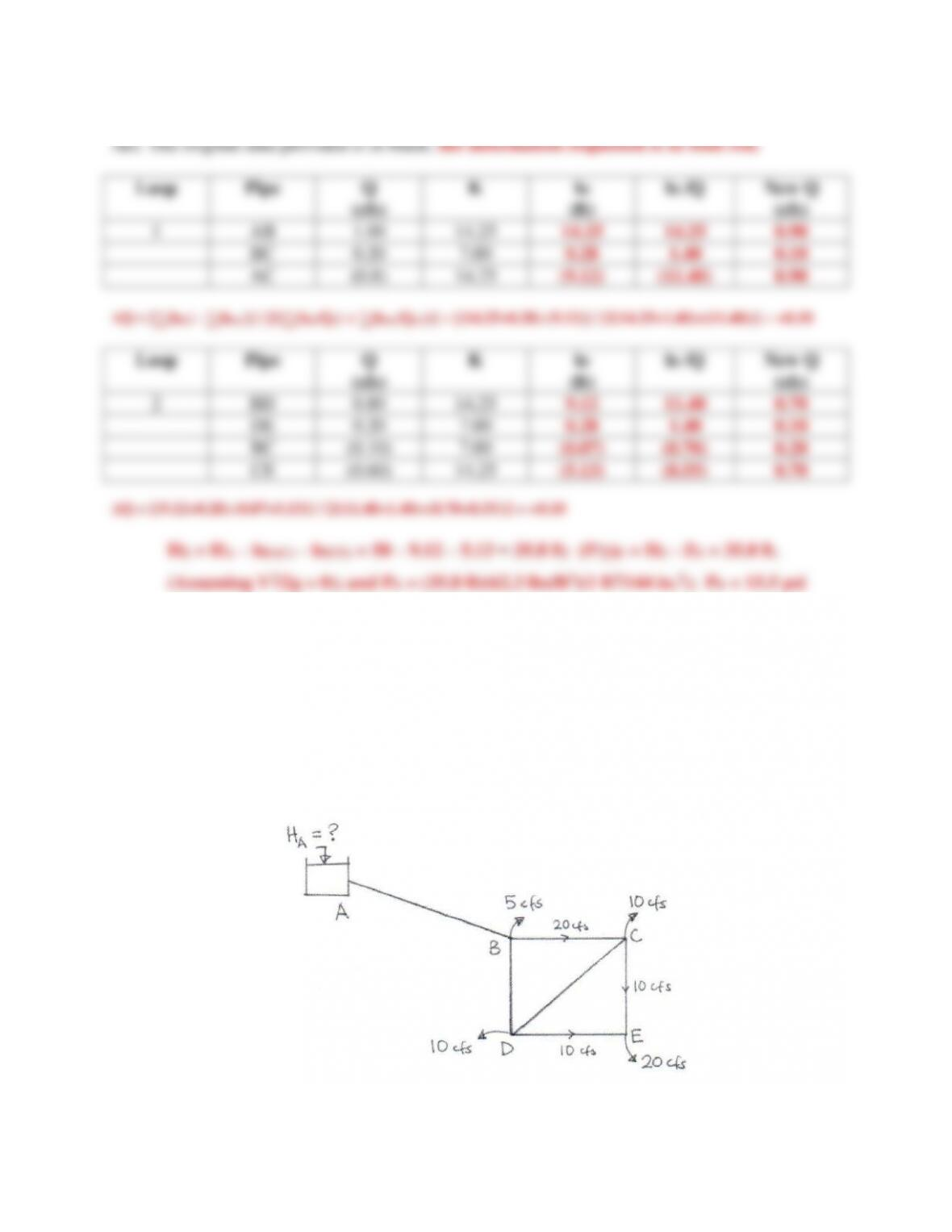41
17. In the pipe network shown below, all of the pipes have a Darcy-Weisbach friction factor of
0.02. Pipe AB is has a length of 5000 ft and a diameter of 3 ft. Each of the pipes BC, BD,
CE, and DE have a length of 1000 ft and a diameter of 2 ft. Pipe DC is 1410 ft long and has a
diameter of 2 ft. The final solution obtained for this network indicates that the flows in pipes
BC, CE, and DE are respectively 20 cfs, 10 cfs, and 10 cfs as shown in the figure. Also, the
energy head at D is 100 feet. Determine the water surface elevation in Reservoir A, the
energy loss in pipe DC, and the energy loss in DC if the diameter were increased to 3 feet.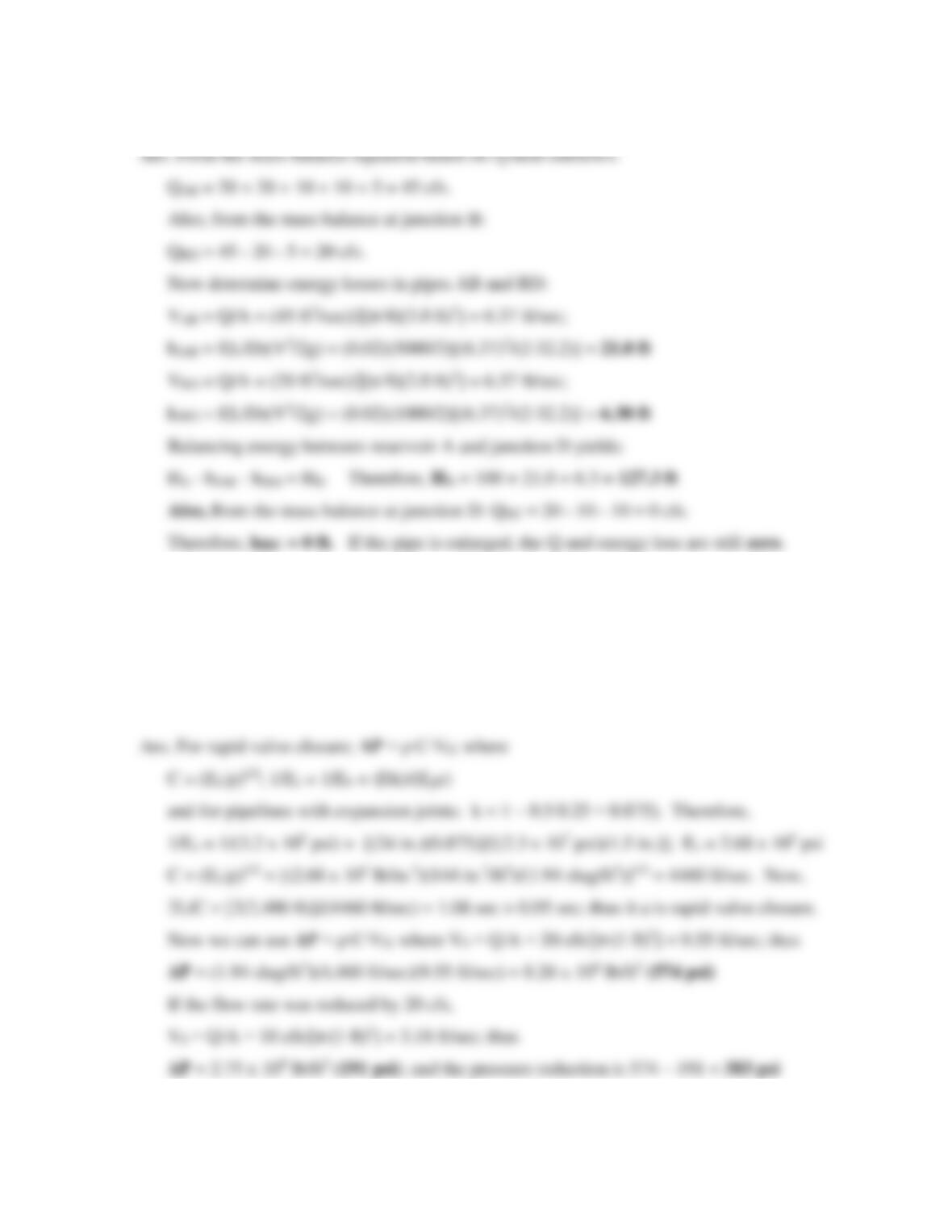42
18. A 2,400-ft-long, 2-ft-diameter pipeline conveys water from a hill-top reservoir to an
industrial site. The pipe is made of ductile iron, has an outside diameter of 2.25 feet, and has
expansion joints. If the flow rate is 30 cfs, determine the maximum water hammer pressure
(in psi) that is likely to occur if the downstream flow valve is closed in 0.95 seconds. Also
determine the water hammer reduction (in psi) if a diverter is added that reduces the flow rate
from 30 cfs to 10 cfs almost instantly upon valve closure.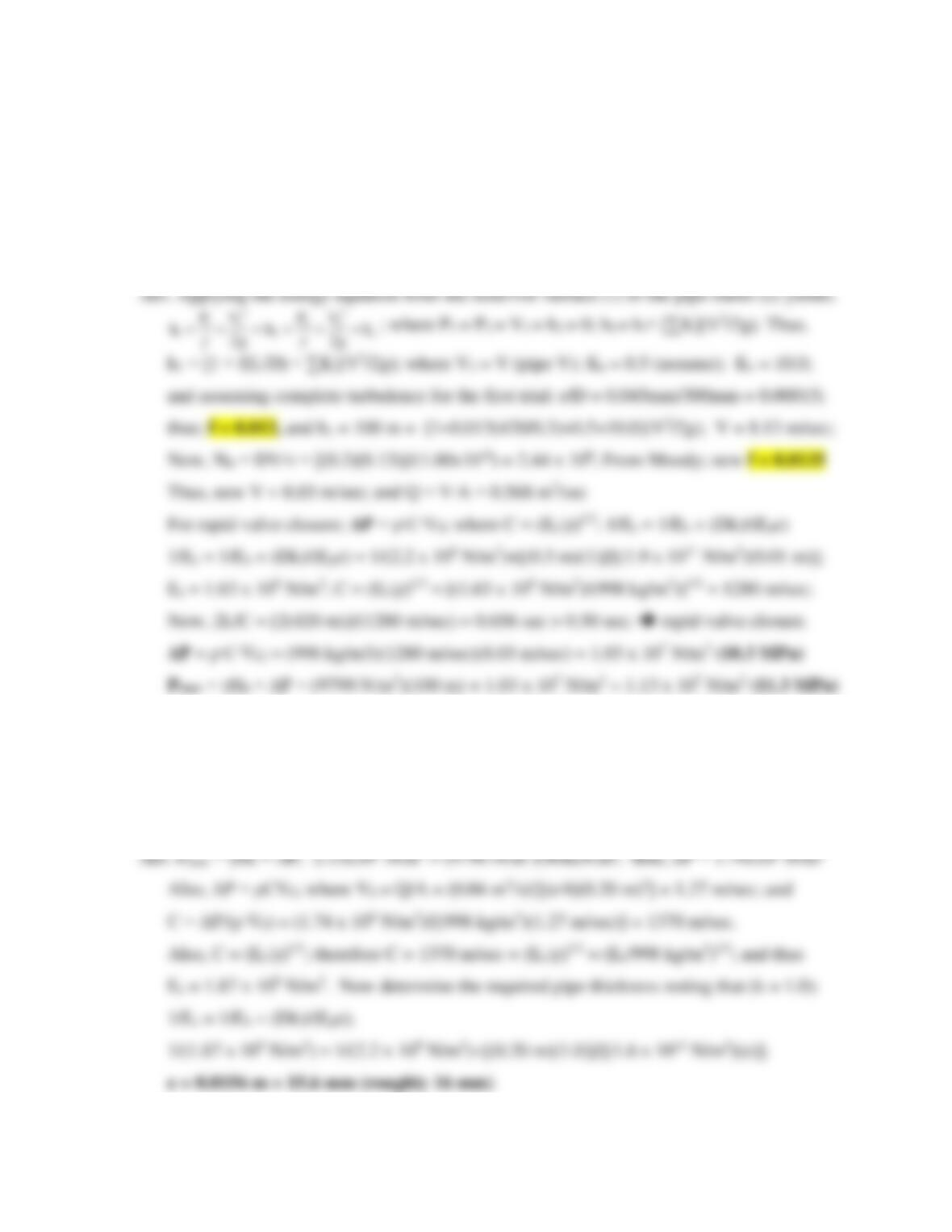43
19. A pipeline conveys water from a reservoir to a point 100 m below its water surface and
discharges into the air. The commercial steel pipe is 30 cm in diameter, 420 m long, has a
wall thickness of 1 cm, and is free to move longitudinally. A rotary valve is installed at the
downstream end. Calculate the maximum water hammer pressure that can be expected on the
valve if it closes in a 0.5-sec period. Also, determine the total (maximum) pressure the
pipeline will be exposed to during the water hammer phenomenon.
20. A pipeline is being designed to withstand a total maximum pressure of 2.13 x 106 N/m2. The
20-cm pipeline is ductile iron and conveys water at 40 liters/sec. Determine the required
thickness of the pipe wall if the operational head on the pipeline is 40 m and it is also subject
to water hammer if the flow control valve on the downstream end is closed suddenly.
Assume that the longitudinal stresses will be negligible when the pipe is installed.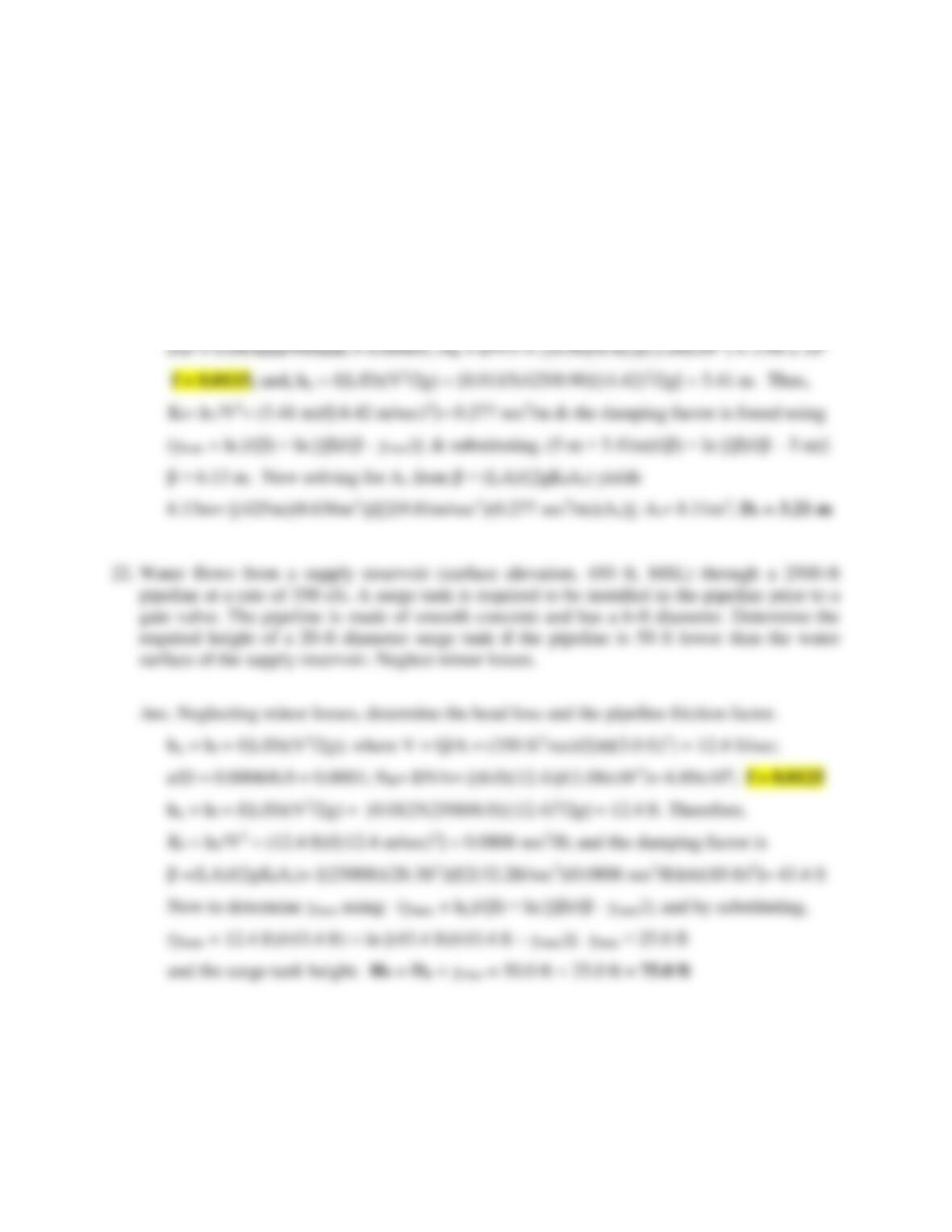44
21. A 425-m-long, commercial steel pipe with a 0.90 m diameter carries irrigation water between
a reservoir and a distribution junction. The maximum flow is 2.81 m3/sec. A simple surge
tank is installed just upstream from the control valve to protect the pipeline from water
hammer damage. Determine the minimum diameter of the surge tank if the allowable water
surface rise is 5 m over the supply reservoir water level. Ignore minor losses.
Ans. Neglecting minor losses, determine the head loss and the pipeline friction factor.
hL = hf = f(L/D)(V2/2g); where V = Q/A = (2.81 m3/sec)/[(π/4)(0.90m)2] = 4.42 m/sec;

## Trusted by Thousands ofStudents

Here are what students say about us.